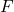# A short tutorial on Bayesian Nonparametrics

Bayesian nonparametric (BNP) models are prior models for infinite-dimensional parameters, such as an unknown probability measureor an unknown regression mean functionWe review some of the most widely used BNP priors, including the Dirichlet process (DP), DP mixture, the Polya tree (PT), and Gaussian process (GP) priors. We discuss how these models are used in typical inference problems. The examples include R code using available packages for inference under BNP priors.

Fulltext